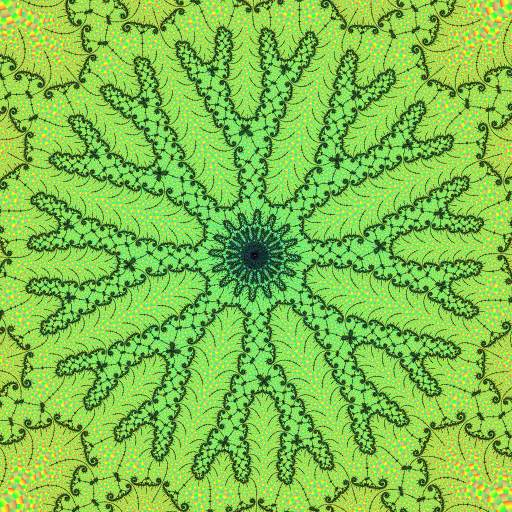Leavitt, Jonathan

Robert P. Munafo, 2009 Nov 7.

A Mandelbrot explorer who has discovered many esoteric and beautiful forms within the higher-order embedded Julia sets, at extremely high magnification using FRACTINT.

Most of his Mandelbrot images are very exotic, including such things as reverse bifurcation. Here is an example:"meta-Zimnila" `^--^Y%.L,^"b,',-'_Yc^` '`F^b/*^^ T *YLc.,:ad/b'r^^L@)- . ._d)br '^(Fv)dF^\ _:-mm,,'.e_/.'_,/'"brdvF^,_:"'\$^^-^^` - a_ c . .dYL'", ./_.'-m/e-.@([-:\$CY\.d@@~\]__`, :^ abFC_-- .- ; FTLL,,` \-/F` ,`"@Fd,^@bT*dF@`;-/b"@@\d^`-/@@_:.L_.,"LL- /.-._a\ "d^d^@mmm"*,^mda:@r@d.-"@c/'@/-^'b-/@^-\':@(@b"^a*- /*"b\)(-@`Ld dL,'['^ -,/ ""@CC"F]L~.^%TC"%b\'d`(@*-`,b@--\$)*^^^-avm^ ''d-v_`: `*m_^---~CYm[c-^*bmvTb,. %*LL'\$/(L(@-'.\\$)c@Y/^;:/Fm/`\_-` .mbeF ^^:@%,:\\$'*7@\/-^`*@.Yb`: .d*@F"_dC^':'/@^/F.:amY^:@mvdC@---/``- .^_@\ad\$F\$(^"%\$\mLC@;"v\;: -,Ya("@c': -@F(@^*"L.d_"""Y^^`^.'@_^. d^*_ -'''^^""rm\$CeL7(`-"FL..-]C-bF. ,)@\`r_mC("C'--_^/.Lv)m--^Y) '__-@@]vd..'_'^```^(^Fe%]7)eaa*('Y__v"-ddF^L/^`..-__/""`mF`,_.-" ``^_'^*d@^b(e_dc/e ,'^`'*b`^L)ba@)d_/m/^`^.- ~.Ld7)d\%%@@-dmL : C,-_rr(F\dmd/r(C\$dve_de__F-b@@@@@@@@@/\mamdr-@^ea/"^"`~^""^"`^%m @b-~^"^^"'~'"^d_)/'`;~^\^r*@@@@@@@@@b)/Lm%r:/-."~bYeLeLeed_Le^*C "--__/eLaL_Lab((^\$F""F(""bF^@@@@@@@@F`FL-'^`^^"))de\$^":\$aFFY[^^% `_d^")F\d/^/_br/T`- ',vmC@b/^F"@F*(\$d:")C;--__'-':'^*FL%@)a,,,.. b' -_r(Lbb*)`~_`--..@m^Fmd("^Tm'dF`FYe)^*@evee%.-._-`,'T*\*` ._ Y@,-^**F^''-.::_m\/^7_"^\$F-'.-@v;@v` `'Ye-\med\d""(-m\a__e`,d:mF ''@-`-._e("")"F"^@vmF"^F-, -\F\~^@\`,`:YC-a(^"^*(e\mF"d\$*,'@7" --\$@`^.*)v*b@()F*^``@Y-@~,`,\d"_e/^Y-d_`:YL`7YF-;`^b"\_`^ ]\$_-- /%T^`_,- ;:FYmF-'m\mY"()\ --aY7:/YmYYLr_`.'LL(^b__d-"Yd"^^-m"(@a `_^'^F.__,'*^``.)/\$\$-\$F- --a\/(F:-7d\$'Cr- /]^*d@(7v,,`/\__,__d%^ _T)F"F^"*\b;.'@C\$"^@(\$"--\$a*_"@F-'(\$\%"b,--'@)b.`("^_\Y*^"Y_La.\ ("".[ '.'-Y7^ '\ \~@bF',\/@(Y_Yb).(@T@mLFe`'/bd`-, .((L` ','.'@d : ' ^^aa*(`__;_,:^` -^@b*,`@[@(-7L\$F,]*F"\_.-__'v""@,__, ', " ----raL^:`./L@)*(Ye_''`` ''^`c''"^`, .'m@*("(CvC_"*\,_^ ` '**^"-'`"*` ` `''"- ` --"**""-- '-~**`' ''"'- "^'*-^--- -1.749078161505201731679124545156633037 + 0.000005509919066290966025130985672062 i @ 1.926e-33, Nmax: 20000

Leavitt created many stunning images from January 1998 to (approximately) 2001. Some of his stunning images could be seen here, but that page no longer works. However the Fractal Foundation still has a few Leavitt images if you look carefully.

A partial snapshot of the old (now-defunct) website at sky-dyes.com can be seen here. Two reverse bifurcation images are here.

My investigation of Leavitt's images in 2002 led to my discovery of the exponential map coordinate transform as a tool for understanding the structure of high-order embedded Julia sets.

revisions: 20080228 oldest on record; 20091107 archive.org is only partial; 20100923 Fractal Foundation website change; 20101205 Add link to exponential map

From the Mandelbrot Set Glossary and Encyclopedia, by Robert Munafo, (c) 1987-2018.     Mu-ency index

mrob27This page was written in the "embarrassingly readable" markup language RHTF, and was last updated on 2018 Feb 04.s.11